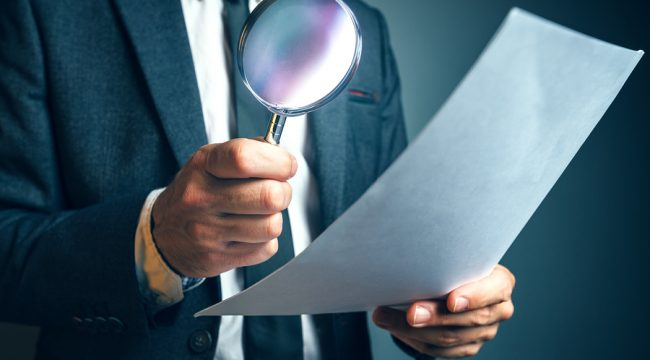# Accounting – 3 Common depreciation methodDepreciation refers to allocating the cost of an asset or referred as “property, plant and equipment” over its useful life. Unfortunately, the cost of an asset may not be uniform over the asset’s lifetime (Also see Accounting tasks to consider before the year ends). Luckily, there are a few depreciation methods that attempt to allocate the cost over the asset’s lifetime. This post looks at the commonly used depreciation methods.

Straight Line Method

The method is straightforward and easy to use. It is defined as the cost of the asset less the residual value (Also see Liquidation Value) divided by the number of years the asset will be useful to the business. Where:

Cost = initial acquisition price

Residual value= the scrap value of the asset

Useful life = the number of years the asset will be used by the business

Every year, the entrepreneur (Also see Accounting – Five mistakes to avoid for business success) must remember to depreciate his asset by subtracting the depreciation figure from the cost of the asset. If an asset depreciates by \$20 per year, the entrepreneur must remember to subtract \$ 20 from the assets book value every year.

Reducing Balance Method

This method charges depreciation (Also see distinguishing depreciation and impairment) at a higher rate at the earlier years of the asset. The amount of depreciation charged reduces over the life time of an asset. To this end, depreciation may be calculated using the formula:

Depreciation = Rate of Depreciation X Asset’s Book value

Where the book value refers to the net value of an asset at the start of the accounting period. The figure is found by deducting the accumulated total from the assets cost. As a result, the asset’s deprecation value for last year can be calculated by getting the differences between the net book value at the start of the period and the residual value. From this figure, it is evident that the value declines progressively as the asset helps the business to generate more profits.

Sum of the Year’s Digits

This method entails calculating depreciation based on the sum of the number of years the asset has been useful. Just like other depreciation methods, the method accelerates depreciation in the first years. The method allocates higher depreciation values in the earlier years of the asset’s useful life. For instance, if the asset is expected to serve the business for 4 years, then the sum of years’ digit will be 4+3+2+1 = 10. Its useful life will then be used to calculate depreciation. It will be four years in the first year, three years in the second year and so forth.

For business owner that is not accounting trained, it is always a good idea to seek help from accounting firm in Johor Bahru if you are not confident to get it done right.